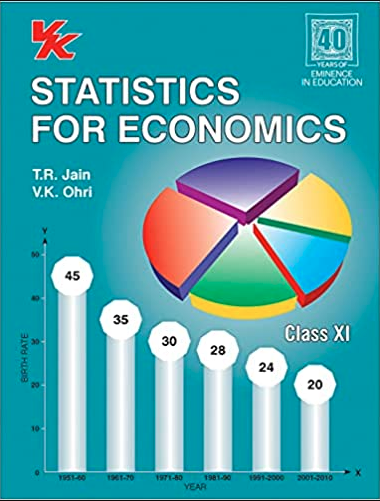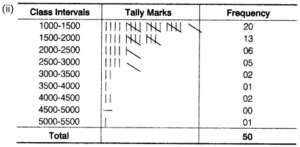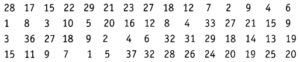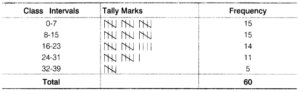# Chapter 3 – Organisation of Data Questions and Answers: NCERT Solutions for Class 11 Economics(Statistics for Economics)

Class 11 Economics(Statistics for Economics) NCERT book solutions for Chapter 3 - Organisation of Data Questions and Answers.## Question 5. Use the data in Table 3.2 that relate to monthly household expenditure (in ₹) on food of 50 households and (i) Obtain the range of monthly household expenditure on food. (ii) Divide the range into appropriate number of class intervals and obtain the frequency distribution of expenditure. (iii) Find the number of households whose monthly expenditure on food is less than ₹ 2,000 more than ₹ 3,000 between ₹ 1,500 and ₹ 2,500

### Answer: (i) Range = Largest Value – Smallest Value Highest Value = 5090 Lowest Value = 1007 So, Range = 5090 – 1007 = 4083## Question 10. Prepare a frequency distribution by inclusive method taking class interval of 7 from the following data